# Simplest form

Find the simplest form of the following expression: 3 to the 2nd power - 1/4 to the 2nd power.

x =  8.9375

### Step-by-step explanation:

$x={3}^{2}-\left(\frac{1}{4}{\right)}^{2}=\frac{143}{16}=8\frac{15}{16}=8.9375$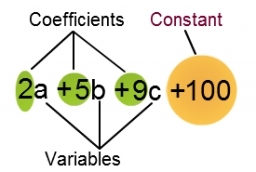Did you find an error or inaccuracy? Feel free to write us. Thank you!Tips to related online calculators
Need help to calculate sum, simplify or multiply fractions? Try our fraction calculator.

## Related math problems and questions:

• Simplest form of a fractionWhich one of the following fraction after reducing in simplest form is not equal to 3/2? a) 15/20 b) 12/8 c) 27/18 d) 6/4
• Fractions and mixed numerals(a) Convert the following mixed numbers to improper fractions. i. 3 5/8 ii. 7 7/6 (b) Convert the following improper fraction to a mixed number. i. 13/4 ii. 78/5 (c) Simplify these fractions to their lowest terms. i. 36/42 ii. 27/45 2. evaluate the follow
• Evaluate mixed expressionsWhich of the following is equal to 4 and 2 over 3 divided by 3 and 1 over 2? A. 4 and 2 over 3 times 3 and 2 over 1 B. 14 over 3 times 2 over 7 C. 14 over 3 times 7 over 2 D. 42 over 3 times 2 over 31
• Evaluate expressionEvaluate following expression: 5 to the power of 2 - (4x4) divided by 2 =
• Square area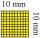Complete the table and then draw each square. Provide exact lengths. Describe any problems you have. Side Length Area Square 1 1 unit2 Square 2 2 units2 Square 3 4 units2
• Simplify 7Simplify. 7-1/3÷ 3-2/3 of 2+ 4-1/2÷ 2-1/4+ 1/2 solution and by step by step
• Athletic race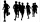In a race, the second-place finisher crossed the finish line 1 1/3 minutes after the first-place finisher. The third-place finisher was 1 3/4 minutes behind the second-place finisher. The third-place finisher took 34 2/3 minutes. How long did the first-pl
• Find the 2Find the term independent of x in the expansion of (4x3+1/2x)8
• Rose spendsRose spends 2 1/3 hours studying Math, 1 3/4 hours studying English, and 2 1/4 hours studying Science. Find her average time studying the three subjects.
• Find unknown 2Find unknown denominator: 2/3 -5/? = 1/4
• Simplify 3Simplify mixed numerals expression: 8 1/4- 3 2/5 - (2 1/3 - 1/4) Show your solution.
• Percent to interval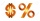76% is between which of the following two numbers. 1) 1/5 & 1/4. 2) 1/3 & 1/2 3) 2/3 & 7/10 4) 3/4 & 5/6
• KingKing had four sons. First inherit 1/2, second 1/4, third 1/5 of property. What part of the property was left to the last of the brothers?
• Sum of 18Sum of two fractions is 4 3/7. If one of the fractions is 2 1/5 find the other one .
• Three digits number 2Find the number of all three-digit positive integers that can be put together from digits 1,2,3,4 and which are subject to the same time has the following conditions: on one positions is one of the numbers 1,3,4, on the place of hundreds 4 or 2.
• Fraction unknowns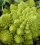Divide of fractions with unknowns: Fraction 1: The quantity x squared plus 6 times x plus 9 over the quantity x minus 1. Fraction 2: the quantity x squared minus 9 over the quantity x squared minus 2 times x plus 1.  Find Fraction 1 over Fraction 2.
• One third power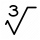Which equation justifies why ten to the one-third power equals the cube root of ten?